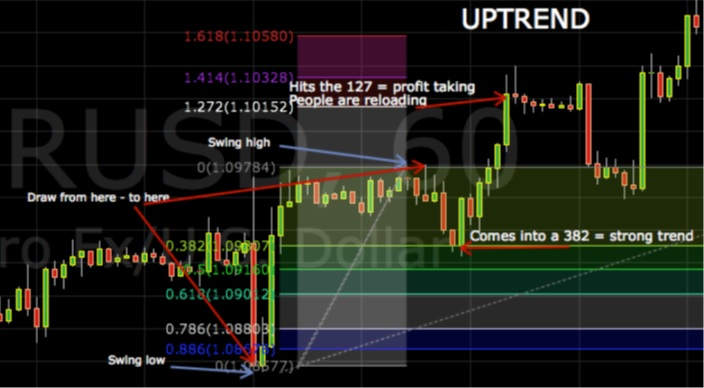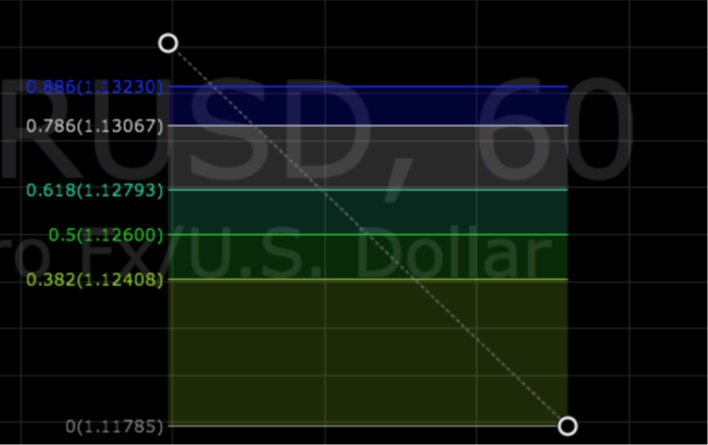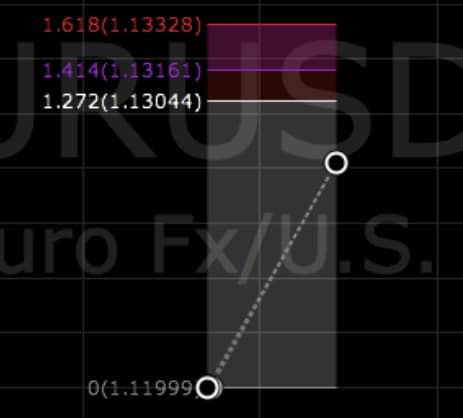You could compare ratio trading to a lot of things in this world to get the complete comprehension of what it is and how it is used. Let’s say that you are an architect and you are planning on drawing the next skyscraper in New York. Even though I do not know the first thing about drawing blueprints or architecture in general, it is safe to say that we all know that architecture does not sit by his desk and willy silly draws something on a piece of paper and expects the builders. Or the people that read his or her “blueprint” to go: “ah yes I see what he is trying to draw here”.

No, an architect uses numbers, math, measurements and more to get his or hers blueprint exactly so that it is possible for the “next” to build off that blueprint. That is what Fibonacci Ratios are; they are a measuring stick, a guide, something that is made up of math, and math formulas. There are more

things that are guaranteed in this world besides death and taxes, math is one of them. The statement above looks to be the answer to all the trading losses in the world, just use Fibonacci ratios, they say that they are guaranteed right? Wrong, Fibonacci is just another tool in our toolbox, it is indeed a mathematically build measuring stick and can be used very beneficial in trading combined with other things. In this lesson, we will go over how to use and draw Fibonacci Ratios.

Fibonacci sequence

Fibonacci was a man, he was a mathematician and was born around 1170, and his real name was Leonardo Pisano. He is best known for his numerical sequence, and we are going to look a little closer into that. The Fibonacci Sequence is the series of numbers:

0, 1, 1, 2, 3, 5, 8, 13, 21, 34, …

The sequence works in a way that you can always take a number and adds it to the previous one to get

the next one in the sequence. So 2+3 = 5, 3+5 = 8, 5+8 = 13 and so on.

Now it starts to become interesting!

OTC derivatives and EMIR

You may have heard about some of the ratios before, like 618, 786 and 382

After the 8th n. in the sequence the magic starts (not magic, just math)

Pull out your calculator’s boys and girls!

55/89 = 0.618

89/144 = 0.618

144/233= 0.618

4181/6765= 0.618

And if we take it the other way!

89/55 = 1.618

144/89 = 1.618

233/144 = 1.618

6765/4181 = 1.618

Well, that can´t be coincident!

You will have to relies upon that these ratios are not there because of trading. These numbers existed long before there was trading or charts, this is what the universe is built on, you can find this in stars, nature and even your ear, hands and fingers, if you start to measure it out, you will see. Now we will take a look at the numbers we use; they are split up into two categories, Primary and Secondary. They are then further broken up into Retracements and Extensions.

Retracements

Primary =             .382 (square of .618)

.618 (Inverse of .1618)

.786 (Inverse sq. root of 1.618)

Secondary =       .50

.886Retracements in use!

So we know now what the levels are, the next step is to learn how to draw them in. Now the basic understanding of structure, swing low – swing high that we learned in our first lessons comes in handy. If you have not given the basics enough time, I suggest you stop reading and go back to the beginning of this course. The way to draw in a Fibonacci level is as follows:

In an uptrend = swing low to swing high

In a downtrend = swing high to swing low

Extensions

Primary = 1.272 and 1.618

Secondary = 1.414Extensions in use!

So we know now what the levels are, the next step is to learn how to draw them in. Now the basic understanding of structure, swing low – swing high that we learned in our first lessons comes in handy. If you have not given the basics enough time, I suggest you stop reading and go back to the beginning of this course. The way to draw in a Fibonacci level is as follows:

In an uptrend = swing low to swing high and back down to swing low

In a downtrend = swing high to swing low and back up into swing high

Fibonacci in action

Let’s take a look at Fibonacci in action on a chart, you can see how to draw them in and see how they work.The purpose

You might be asking yourselves well, that’s all fine and dandy in hinge sight but when stepping into the real deal, what does this mean?

Retracement = where the market is likely to stop, pause after profit taking and or some selling/buying pressure.

Extensions = where the market is likely to go, then take a pause and continue, or reverse. In trend, this is a rule of thumb (not a rule, good to know)

The 382 ret = Strong trend

The 1.618 ext. = The golden mean (phi)

At last, I feel the need to encourage you not to take this as some magic tool and go out and start trading all the retracements and extensions you find. Remember Fibonacci is just another tool in our ever growing toolbox, it’s getting larger, and you are becoming better (I hope).